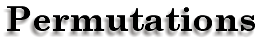Sometimes it's not so easy to figure out the number of possible outcomes, like when you have several items with a number of choices. The Counting Principle can help. With the Counting Principle, all you need to do is multiply the number of choices for each item.For instance:You have 2 different types of rings, 4 types of hats, and 3 jackets.How many combinations can you make?Multiply the number of watches by the number of hats, 3x4=12. Then multiply that by the number of jackets, 12x2=24. There are 24 possible combinations.That's all it is. And that took a lot less time than trying on 24 different outfits, didn't it?Independent and Dependent Events
 writing: Kirsten Anderson art: mC